## Friday, July 31, 2015

### Simulating an arbitrary shift in the diversification rate on a phylogeny

A R-sig-phylo reader recently asked if it is possible to simulate a shift in the rate of lineage diversification in some part of a tree (rather than simultaneously across all the lineages in a tree at a given time).

Now, I'll first say that I understand that methods to simulate various state-based models of diversification exist in the diversitree R package maintained by Rich Fitzjohn. If, however, one just wants to arbitrarily pick a point in the tree & switch to a different diversification rate (speciation and/or extinction rate) then this can also be done by merely simulating a complete tree under one process, going to the spot where you want the rate to change, pruning out the subtree tipward of that point, simulating a new subtree under your new desired process, and the pasting the two trees together.

Since this is easier said than done in R, the code below shows an example in which I first simulate a base tree with a birth rate of about `b~0.02`, a taxon-stop criterion of `20` and a time stop of `100`; then I (arbitrarily, just to demonstrate) pick a random lineage at 75% of the total tree height and call that my shift point; I prune that lineage out, and attach a new subtree in which I use a much higher birth-rate of about `b~0.12`.

Here's what that looks like:

``````library(phytools)
## set the birth rate based on n=20 & t=100
b1<-(log(20)-log(2))/100
b1
``````
``````##  0.02302585
``````
``````tree<-pbtree(b=b1,t=100,n=20)
``````
``````## simulating with both taxa-stop (n) and time-stop (t) is
## performed via rejection sampling & may be slow
##
##   4 trees rejected before finding a tree
``````
``````plotTree(tree,mar=c(3.1,0.1,0.1,0.1))
axis(1)
nodelabels()
``````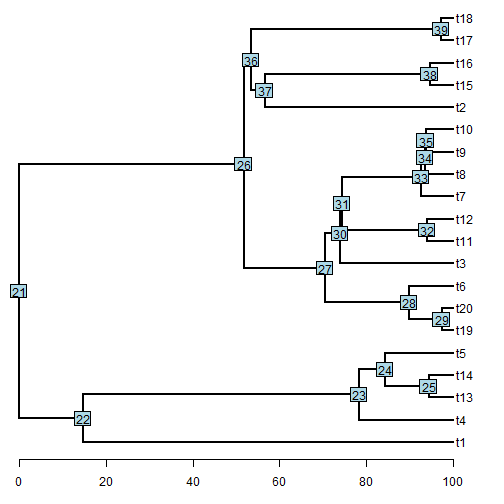``````## pick a split point at 75% of total tree height
pp<-0.75
H<-nodeHeights(tree)
node<-sample(tree\$edge[(H[,1]<pp*max(H))+
(H[,2]>pp*max(H))==2,2],1)
node
``````
``````##  28
``````
``````## split the tree & this point
tree<-splitTree(tree,split=list(node=node,
bp=pp*max(H)-H[which(tree\$edge[,2]==node),1]))[]
tree\$tip.label[tree\$tip.label=="NA"]<-""
plotTree(tree,mar=c(3.1,0.1,0.1,0.1))
axis(1)
nodelabels(node=tree\$tip.label=="",pch=19)
``````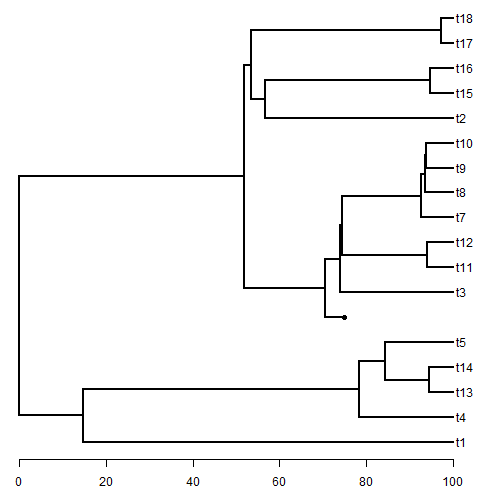``````## set the second desired birth-rate based on n=25 & t=25
## note this time we want to start with 1 lineage, not 2
b2<-log(25)/(max(H)-pp*max(H))
## simulate the "stem" of the new subtree
t1<-(max(H)-pp*max(H))
while(t1>=25) t1<-rexp(n=1,rate=b2)
ii<-which(tree\$edge[,2]==which(tree\$tip.label==""))
tree\$edge.length[ii]<-tree\$edge.length[ii]+t1
plotTree(tree,mar=c(3.1,0.1,0.1,0.1))
axis(1)
nodelabels(node=tree\$tip.label=="",pch=19)
``````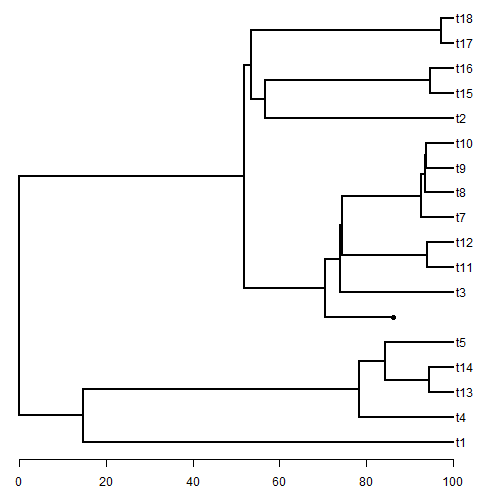``````## now simulate the rest of the derived subtree
t2<-pbtree(b=b2,t=max(nodeHeights(tree))-
nodeheight(tree,which(tree\$tip.label=="")),n=25)
``````
``````## simulating with both taxa-stop (n) and time-stop (t) is
## performed via rejection sampling & may be slow
##
##   103 trees rejected before finding a tree
``````
``````t2\$tip.label<-paste("s",1:Ntip(t2),sep="")
tree<-bind.tree(tree,t2,where=which(tree\$tip.label==""))
plotTree(tree,fsize=0.8,mar=c(3.1,0.1,0.1,0.1))
axis(1)
``````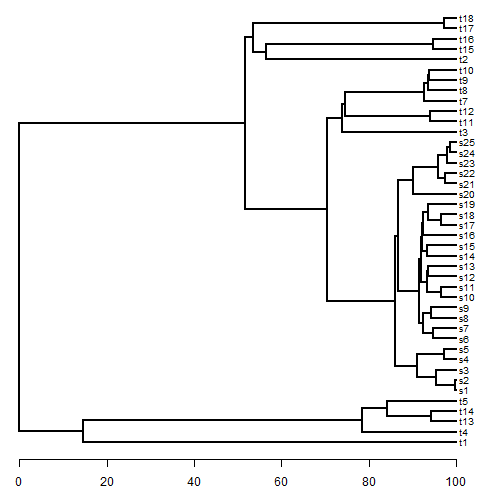``````## visualize the shift in diversification rate
ltt(tree,show.tree=TRUE)
``````
``````## Object of class "ltt" containing:
##
## (1) A phylogenetic tree with 42 tips and 41 internal nodes.
##
## (2) Vectors containing the number of lineages (ltt) and branching times (times) on the tree.
##
## (3) A value for Pybus & Harvey's "gamma" statistic of 2.8428, p-value = 0.0045.
``````
``````obj<-get("last_plot.phylo",envir=.PlotPhyloEnv)
points(x=pp*max(H),y=obj\$yy[findMRCA(tree,t2\$tip.label)],pch=19)
lines(rep(pp*max(H),2),par()\$usr[3:4],lty="dashed")
``````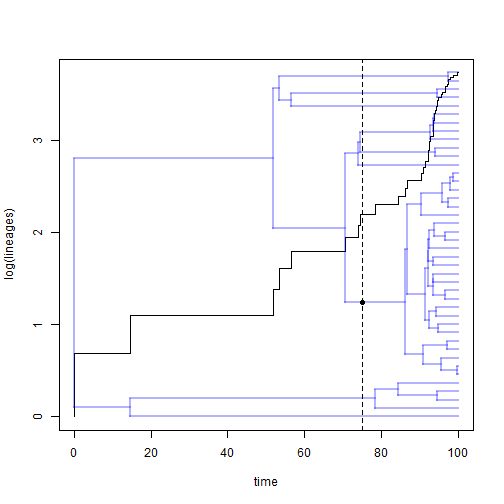That's it.

Obviously, this would have to be customized to whatever scenario the user is envisioning.

#### 1 comment:

1.Een zeer stijlvol replica IWC horloge, dit is een prachtig geschenk. Het ziet er zeer solide uit, het heeft een bepaald gewicht.Tips voor het kopen van een replica hier, IWC horloges kopiëren Er zijn veel horloges met replica's van beroemde designermerken waar we zeker van zijn dat het van hoge kwaliteit en uiterlijk is.

Note: due to the very large amount of spam, all comments are now automatically submitted for moderation.